# Even Number Series Program in Java

In this tutorial you will learn about the Even Number Series Program in Java and its application with practical example.

## Even Number Series Program in Java

In this tutorial, we will learn to create a Java program that will Print Even Number Series using Java programming.

## Prerequisites

Before starting with this tutorial, we assume that you are the best aware of the following C programming topics:

• Operators in Java Programming.
• Basic Input and Output function in Java Programming.
• Basic Java programming.
• For loop in Java programming.
• Conditional Statements in Java programming.
• Arithmetic operations in Java Programming.

## What is an Even number?

A number is said to be an Even number when that number is divided by two into two equal whole numbers. And there should be a remainder of zero when it is being divided by 2.

## Program to Print Even Numbers from 1 to N.

In this program, we will first take the input point from the user. Then we will find the Even numbers up to that range. At last, we will print that series of Even numbers up to that number.

With the help of this program, we can print a series of Even numbers up to N number.

## Output:-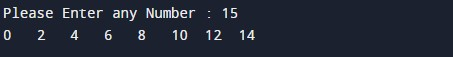In the above program, we have first initialized the required variable.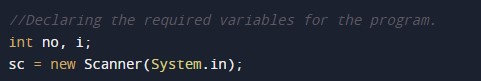• no = it will hold the integer value of the input.
• i = it will hold the integer value for the loop.

Input message for the user to take an endpoint of the series.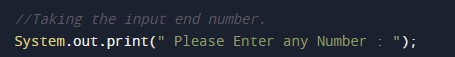Program Logic Code to generate the series of Even numbers.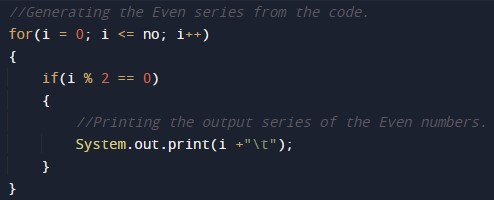Printing output Even digits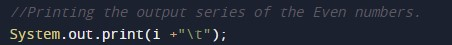In this tutorial we have learn about the Even Number Series Program in Java and its application with practical example. I hope you will like this tutorial.